# Data Types in C++

## Data Types in C++:

Let us talk about Data Types in C++. Data types are very important. See we write the programs (the instructions) so that they can perform some operations on the data. So without data, there is no need for programs. The question is where do you keep the data in your programs? We keep the data in variables in the programs. We can select the data type for a variable depending on the type of value that we want to store in a variable. There are predefined data types available in C++. Just like in any programming language, there will be some predefined data types so here C++ also we have built-in data types available.

We have a table that shows all the data types that are available in C++.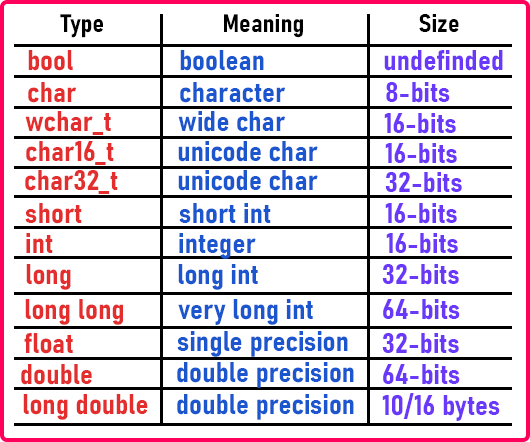We can categorize these data types into 3 types.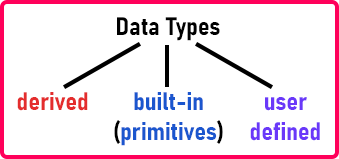The built-in or primitive data type is further divided into 2 types.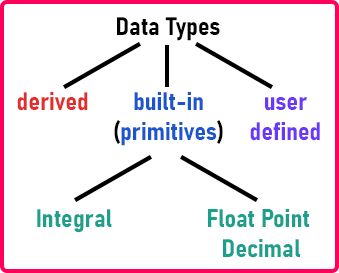This categorization is without a decimal point and with a decimal point. Now inside the integral, we have int and char types and inside the float, we have float and double types.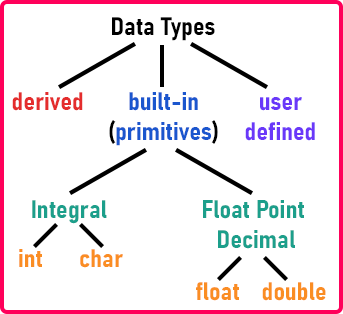Shortintlong and long long are all integer types. Charwchar_tchar16_t, and char32_t are all character types. Float is of float type and double and long double are of double types. The last type is a bool that is of Boolean type. There is one more data type that we have not mentioned in the above table which is the void type.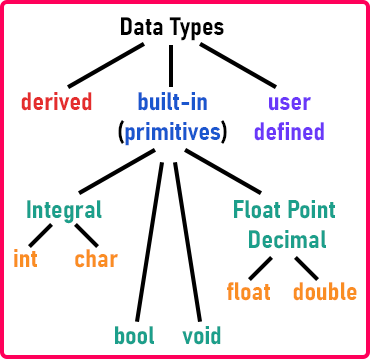##### What are Derived Data Types?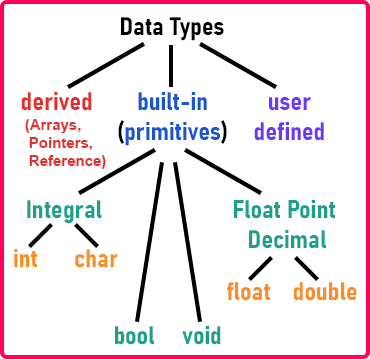Derived data types are arrays, pointers, and References. These are derived data types. Using the primitive data types only we can extend them and form the derived data types.

In user-defined data types, we can define our own data types by using structure, class, enum, and union. So we have listed all possible data types in C++.

Now we will take all the data types from the above table and explain them. The size of the data type depends on the compiler and the operating system on which it is developed. Different compilers of C++ give different sizes for these data types. So in the above table, we have written the minimum number of bits that each data type takes. It may take more than that.

Let us talk about the character data type. Char is useful for storing a character. Character means any English language alphabet. So all the characters that are there on the keyboard come under the English alphabet i.e. character set. So we can store any character and the char data type is useful for storing just one character. Char data type takes 8 bits. But the question is our machines or computers cannot understand the English alphabet. They work on binary number systems. The alphabet should also be converted into a number. So there are some numeric codes defined for these characters. Those codes are known as ASCII codes.

##### ASCII – American Standard Code for Information Interchange.

So some important ASCII codes are as follows: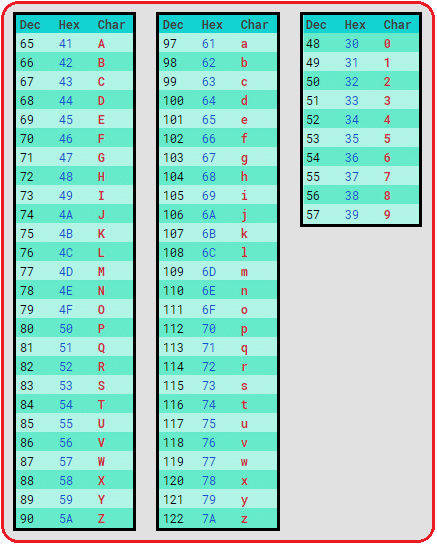###### Symbol ASCII Code

A to Z: 65 to 90
a to z: 97 to 122
0 to 9: 48 to 57

These are some important ASCII codes so you should remember these ASCII codes. You should be able to quickly recall the codes for any characters. For special characters also, ASCII codes are available.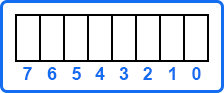The size of the char data type is 8 bits or 1 byte. So in 1 byte, it can store the ASCII code for any of the characters in binary form. Suppose we have to store ‘A’ in char. The ASCII code of ‘A’ is 65 and the binary form is 1000001. To store 65 (i.e. A) in the memory, the binary digits will be written as,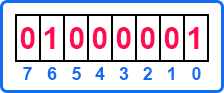So it is not storing ‘A’. It is storing a numeric value (i.e. 65). That’s why we say that character is also an integral type. As the data type is the character so it is interpreted as a character alphabet. If you want to display it on the screen then it will show ‘A’ instead of 65.

Now one more thing about char, if we don’t see it as a character and see it as a number then in 8 bits, what is the minimum and maximum number that it can store? This is a very important thing that one must know.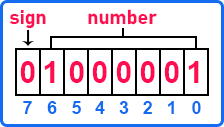We know that, in 8 bits, one bit is reserved as a sign bit and the number is stored in the remaining 7 bits. But how characters can have a sign? Character is also taken as an integral type of data. This is the reason for its negative as well as positive values. So in 7 bits, what is the maximum number that we can store?

27 = 128, but as we are starting from 0 so the range will be 0 to 127. So it means, in 7 bits, the min and max numbers are,

0000000 – 0 (minimum)
1111111 – 127 (maximum)

So within this 1 byte, we can store a number from -127 to 127. So sign bit can be either 0 or 1. 0 means it is positive and 1 means it is negative. But 0 cannot be negative. So we call negative 0 as -128. So the final range of min and max number is from -128 to 127

We have more types of char available in C++ i.e. wchar_t, char16_t, char32_t. wchar_t is a wide character that takes 16 bits. This is useful for storing large-size characters. Character act as an integral. So wchar_t is useful for storing a large-size integer. Char16_t and char32_t data types are useful for supporting Unicode. Because of the internet, natural language support is growing. So you will find that the websites are available in various natural languages i.e. Chinese, Japanese, and Hindi. So in the same way, a program also uses those languages. So for various natural languages, the codes are available and those codes are known as Unicode. To support those natural languages, these data types are available in C++.

If you want to know about Unicode then you can visit the website – Unicode.org. There you can find out the code for various natural languages. This Unicode may not be supported on every C++ compiler but the data types are available.

##### Data Types in C++In integer, we have an int data type that takes 16 bits or 2 bytes. Short is also an integer type and it takes 16 bits. By default, an int itself means a short int. You can make an integer of long type and it takes 32 bits or 4 bytes. You can use long to store larger size values. And also, there is a long long type available in C++ that takes 64 bits or 8 bytes.

Now one important thing is that we all are using 64 bits machines. Before that 32 bits machines were in use. And before earlier to that 16 bits machines were there. So mostly in the languages like C/C++ the size of the integer depends on the bit size of the machine. If it is 16 bits machine then the integer will take 16 bits or 2 bytes. If the machine is 64 bits then the integer will take 64 bits or 8 bytes. The minimum size that an integer takes in C++ is 16 bits. C++ was introduced when 16 bits machines were existing. So that’s why the minimum size of the integer is 16 bits then later 32 bits and 64 bits machines are introduced so the size of the integer is extended to 64 bits. So the size of an integer depends on the word size of the machine. Word size means the bit capacity of a machine.

Float always takes 4 bytes and character takes 1 byte but the size of the integer is depend on the bit size.

Now let us understand how a number is stored in 16 bits.

What is the maximum and minimum number we can store in 16 bits? Let us see.

216 = 65536

0 to 65536

But out of 16 bits, one bit is reserved as a sign bit, and the remaining 15 bits are used for storing a number.Now in 15 bits,

215 = 32768, so the range is 0 to 32768. Including positive and negative numbers the range is -32768 to 32767. This is the range that can be stored in 16 bits. The minimum number you can store in 16 bits is -32768 and the maximum is 32767. As a programmer, you must know this range.

Now one more interesting thing we have to show you is that one programmer must know this. So let us talk about negative numbers. Let us see how the negative numbers are stored. We know that if the sign bit is 0 then the number is positive and for 1 the number is negative. Let us take a number and show you.

The number we want to store is 10. The binary form of 10 is 1010. And all the remaining bits will be zero.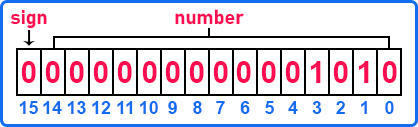This is how 10 is stored in 16 bits. Now if we want to store -10, we have to complement the binary form of 10. It means all the zeros will become 1 and ones become 0. So this will be,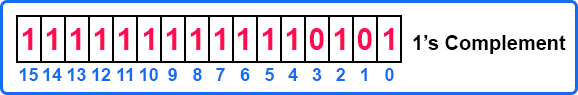This is complemented form so we call it 1’s complement. Now we have to add 1 to this binary number. The result will be,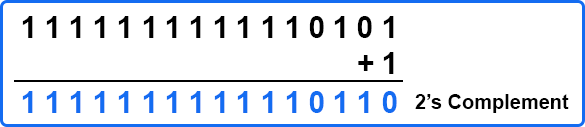After adding 1 to the 1’s complement, we have got the 2’s complement. In most programming languages, a negative number is stored n 2’s complement form. So what is 2’s complement form? When the binary form of a number is complemented then it is known as 1’s complement form. And when 1 is added to 1’s complement then it is known as 2’s complement. So decimal form of 1111111111110110 is -10.

So negative numbers are stored in 2’s complement form. So we have learned the negative number representation. This is common for character and integer data types. Now let us discuss float and double data types.

Float and double data types support decimal numbers. Suppose we have a decimal number 16.34. So the decimal point is supported by the only float and double types. Why it is called float? See if the number is stored with the decimal point between 6 and 3 then it is called a fixed point decimal. But there is a scientific representation or there is another method available for representing a decimal that is called a floating point.

So what we will do? We will move the point to the beginning digit. Suppose we have 16.34, so will shift the point to 0.1634 X 102. So this is a floating point. That’s why we call it float. We can also move the point after the last digit as 1634. X 10-2. So it can be done either way but there is some standard followed in the binary form, not in the decimal form. The standard is called IEEE 754 standard. This is a standard available for floating point numbers. Every electronic device and every programming language follows the same method for representing a decimal number which is floating point representation. You can study this standard by yourself. We have just given you an idea in the decimal form not in binary form. So let us understand from decimal only.

.1624 X 102

See, if we follow this method and the decimal is before the first digit then we don’t have to write the decimal before the first digit. We can simply write,

1624E2

Here “1624” is called mantissa and “2” is called Exponent. So floating point number is stored in two parts that are mantissa and exponent.

16.24

Otherwise, if you follow the fixed point decimal then “16” is called an integral part and “24” is called a decimal part. If we use the above method (mantissa and exponent) then we can store large numbers. This is the scientific notation. If you know more about this then you can study it by yourself.

If you want a more precise number that is a high precision number then you can use a double data type. It is the same as float but the precision is high. And long double has much higher precision than a double. So you can choose according to your need.

Now the next data type is bool. Bool is for Boolean. Boolean values are either true or false. True is taken as 1 and false is taken as 0. Basically, false means 0, and any other number except 0 is taken as true. So it means -10, 14, -14432 or any other number except 0 means true. This bool data type is like integer type only. So it may take either 1 byte or 2 bytes. So that’s why it is undefined. It depends on the compiler. It will store only integer values but not negative values. So we can say 0 means false and 1 or any other number means true. This will improve the readability of the program if you declare this type of variable in your program.

One more data type remaining that we have not listed in the above table is void. Actually, a void data type is there but you cannot declare a variable of the void type. Void means nothing. Then what is the use of void? We can declare a pointer of type void.

Void *ptr.

That’s all about data types. Try to memorize these things which we have discussed in this article. In the next article, I am going to discuss the Size and Range of Data Types in C++ with Examples.